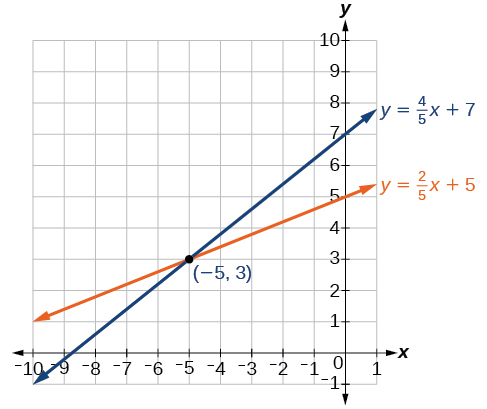# 7.1 Systems of linear equations: two variables  (Page 2/20)

 Page 2 / 20

Another type of system of linear equations is an inconsistent system , which is one in which the equations represent two parallel lines. The lines have the same slope and different y- intercepts. There are no points common to both lines; hence, there is no solution to the system.

## Types of linear systems

There are three types of systems of linear equations in two variables, and three types of solutions.

• An independent system    has exactly one solution pair $\text{\hspace{0.17em}}\left(x,y\right).\text{\hspace{0.17em}}$ The point where the two lines intersect is the only solution.
• An inconsistent system    has no solution. Notice that the two lines are parallel and will never intersect.
• A dependent system    has infinitely many solutions. The lines are coincident. They are the same line, so every coordinate pair on the line is a solution to both equations.

[link] compares graphical representations of each type of system.

Given a system of linear equations and an ordered pair, determine whether the ordered pair is a solution.

1. Substitute the ordered pair into each equation in the system.
2. Determine whether true statements result from the substitution in both equations; if so, the ordered pair is a solution.

## Determining whether an ordered pair is a solution to a system of equations

Determine whether the ordered pair $\text{\hspace{0.17em}}\left(5,1\right)\text{\hspace{0.17em}}$ is a solution to the given system of equations.

$\begin{array}{l}\text{\hspace{0.17em}}x+3y=8\hfill \\ \text{\hspace{0.17em}}2x-9=y\hfill \end{array}$

Substitute the ordered pair $\text{\hspace{0.17em}}\left(5,1\right)\text{\hspace{0.17em}}$ into both equations.

The ordered pair $\text{\hspace{0.17em}}\left(5,1\right)\text{\hspace{0.17em}}$ satisfies both equations, so it is the solution to the system.

Determine whether the ordered pair $\text{\hspace{0.17em}}\left(8,5\right)\text{\hspace{0.17em}}$ is a solution to the following system.

$\begin{array}{c}5x-4y=20\\ \text{\hspace{0.17em}}\text{\hspace{0.17em}}\text{\hspace{0.17em}}2x+1=3y\end{array}$

Not a solution.

## Solving systems of equations by graphing

There are multiple methods of solving systems of linear equations. For a system of linear equations    in two variables, we can determine both the type of system and the solution by graphing the system of equations on the same set of axes.

## Solving a system of equations in two variables by graphing

Solve the following system of equations by graphing. Identify the type of system.

$\begin{array}{c}2x+y=-8\\ \text{\hspace{0.17em}}\text{\hspace{0.17em}}\text{\hspace{0.17em}}x-y=-1\end{array}$

Solve the first equation for $\text{\hspace{0.17em}}y.$

$\begin{array}{c}2x+y=-8\\ \text{\hspace{0.17em}}\text{\hspace{0.17em}}\text{\hspace{0.17em}}\text{\hspace{0.17em}}\text{\hspace{0.17em}}\text{\hspace{0.17em}}\text{\hspace{0.17em}}\text{\hspace{0.17em}}\text{\hspace{0.17em}}\text{\hspace{0.17em}}\text{\hspace{0.17em}}\text{\hspace{0.17em}}\text{\hspace{0.17em}}\text{\hspace{0.17em}}\text{\hspace{0.17em}}\text{\hspace{0.17em}}\text{\hspace{0.17em}}\text{\hspace{0.17em}}\text{\hspace{0.17em}}\text{\hspace{0.17em}}\text{\hspace{0.17em}}\text{\hspace{0.17em}}\text{\hspace{0.17em}}\text{\hspace{0.17em}}\text{\hspace{0.17em}}\text{\hspace{0.17em}}y=-2x-8\end{array}$

Solve the second equation for $\text{\hspace{0.17em}}y.$

$\begin{array}{c}x-y=-1\\ \text{\hspace{0.17em}}\text{\hspace{0.17em}}\text{\hspace{0.17em}}\text{\hspace{0.17em}}\text{\hspace{0.17em}}\text{\hspace{0.17em}}\text{\hspace{0.17em}}\text{\hspace{0.17em}}\text{\hspace{0.17em}}\text{\hspace{0.17em}}\text{\hspace{0.17em}}\text{\hspace{0.17em}}\text{\hspace{0.17em}}\text{\hspace{0.17em}}\text{\hspace{0.17em}}y=x+1\end{array}$

Graph both equations on the same set of axes as in [link] .

The lines appear to intersect at the point $\text{\hspace{0.17em}}\left(-3,-2\right).\text{\hspace{0.17em}}$ We can check to make sure that this is the solution to the system by substituting the ordered pair into both equations.

The solution to the system is the ordered pair $\text{\hspace{0.17em}}\left(-3,-2\right),$ so the system is independent.

Solve the following system of equations by graphing.

The solution to the system is the ordered pair $\text{\hspace{0.17em}}\left(-5,3\right).$Can graphing be used if the system is inconsistent or dependent?

Yes, in both cases we can still graph the system to determine the type of system and solution. If the two lines are parallel, the system has no solution and is inconsistent. If the two lines are identical, the system has infinite solutions and is a dependent system.

how do I set up the problem?
what is a solution set?
Harshika
find the subring of gaussian integers?
Rofiqul
hello, I am happy to help!
Abdullahi
hi mam
Mark
find the value of 2x=32
divide by 2 on each side of the equal sign to solve for x
corri
X=16
Michael
Want to review on complex number 1.What are complex number 2.How to solve complex number problems.
Beyan
yes i wantt to review
Mark
use the y -intercept and slope to sketch the graph of the equation y=6x
how do we prove the quadratic formular
Darius
hello, if you have a question about Algebra 2. I may be able to help. I am an Algebra 2 Teacher
thank you help me with how to prove the quadratic equation
Seidu
may God blessed u for that. Please I want u to help me in sets.
Opoku
what is math number
4
Trista
x-2y+3z=-3 2x-y+z=7 -x+3y-z=6
can you teacch how to solve that🙏
Mark
Solve for the first variable in one of the equations, then substitute the result into the other equation. Point For: (6111,4111,−411)(6111,4111,-411) Equation Form: x=6111,y=4111,z=−411x=6111,y=4111,z=-411
Brenna
(61/11,41/11,−4/11)
Brenna
x=61/11 y=41/11 z=−4/11 x=61/11 y=41/11 z=-4/11
Brenna
Need help solving this problem (2/7)^-2
x+2y-z=7
Sidiki
what is the coefficient of -4×
-1
Shedrak
the operation * is x * y =x + y/ 1+(x × y) show if the operation is commutative if x × y is not equal to -1
An investment account was opened with an initial deposit of \$9,600 and earns 7.4% interest, compounded continuously. How much will the account be worth after 15 years?
lim x to infinity e^1-e^-1/log(1+x)
given eccentricity and a point find the equiationByByBy Cath YuBy Brooke DelaneyBy Brooke DelaneyByBy Tony PizurBy Jordon HumphreysBy OpenStaxBy OpenStaxBy Madison ChristianBy Saylor Foundation